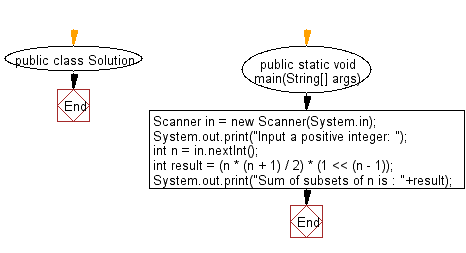﻿ Java programming exercises: Sum of all the elements from all possible subsets of a set formed by first n natural numbers - w3resource# Java Exercises: Sum of all the elements from all possible subsets of a set formed by first n natural numbers

## Java Basic: Exercise-193 with Solution

Write a Java program that accept an integer and find the sum of all the elements from all possible subsets of a set formed by first n natural numbers.

Sample Solution:

Java Code:

``````import java.util.Scanner;
public class Solution {
public static void main(String[] args) {
Scanner in = new Scanner(System.in);
System.out.print("Input a positive integer: ");
int n = in .nextInt();
int result = (n * (n + 1) / 2) * (1 << (n - 1));
System.out.print("Sum of subsets of n is : " + result);
}
}
```
```

Sample Output:

```Input a positive integer:  25
Sum of subsets of n is : 1157627904
```

Flowchart:Java Code Editor:

Company:

What is the difficulty level of this exercise?

﻿

## Java: Tips of the Day

Parsing dates:

```import java.io.*;
import java.util.*;
import java.text.*;

String s = "2001/09/23 14:39";

SimpleDateFormat formatter = new SimpleDateFormat ("yyyy/MM/dd H:mm");
Date d = formatter.parse(s, new ParsePosition(0));
```